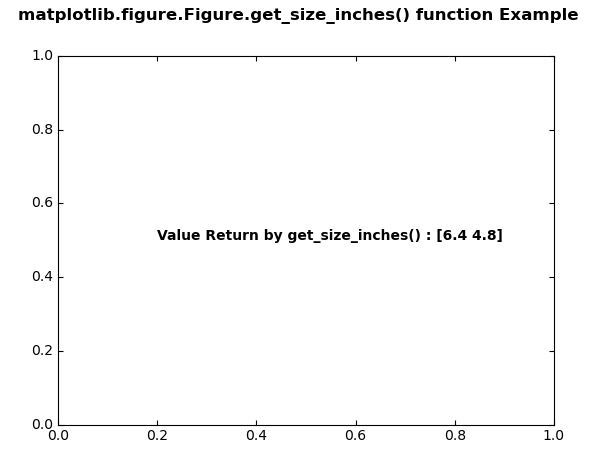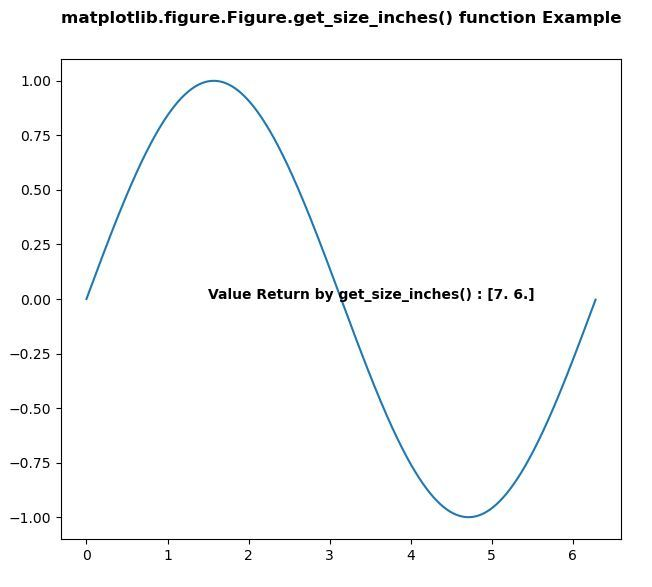# Matplotlib.figure.Figure.get_size_inches() in Python

Matplotlib is a library in Python and it is numerical – mathematical extension for NumPy library. The figure module provides the top-level Artist, the Figure, which contains all the plot elements. This module is used to control the default spacing of the subplots and top level container for all plot elements.

## matplotlib.figure.Figure.get_size_inches() method

The get_size_inches() method figure module of matplotlib library is used to get the current size of the figure in inches.

Syntax: get_size_inches(self)

Parameters: This method does not accept any parameters.

Returns: This method return size (width, height) of the figure in inches.

Below examples illustrate the matplotlib.figure.Figure.get_size_inches() function in matplotlib.figure:

Example 1:

 `# Implementation of matplotlib function  ` `import` `matplotlib.pyplot as plt  ` `from` `matplotlib.figure ``import` `Figure ` `from` `mpl_toolkits.axisartist.axislines ``import` `Subplot  ` `import` `numpy as np  ` `    `  `fig ``=` `plt.figure()  ` `     `  `ax ``=` `Subplot(fig, ``111``)  ` `fig.add_subplot(ax)   ` ` `  `w ``=` `fig.get_size_inches() ` `ax.text(``0.2``, ``0.5``, ` `        ``"Value Return by get_size_inches() : "` `        ``+` `str``(w), ` `        ``fontweight ``=``"bold"``) ` `   `  `fig.canvas.draw() ` ` `  `fig.suptitle('matplotlib.figure.Figure.get_size_inches()\ ` ` ``function Example', fontweight ``=``"bold"``)  ` ` `  `plt.show() `

Output:Example 2:

 `# Implementation of matplotlib function  ` `import` `matplotlib.pyplot as plt  ` `from` `matplotlib.figure ``import` `Figure ` `import` `numpy as np  ` `      `  `fig ``=` `plt.figure(edgecolor ``=` `"red"``, figsize ``=``(``7``, ``6``))  ` `      `  `ax ``=` `fig.add_axes([``0.1``, ``0.1``, ``0.8``, ``0.8``]) ` `    `  `xx ``=` `np.arange(``0``, ``2` `*` `np.pi, ``0.01``)  ` `ax.plot(xx, np.sin(xx))  ` `  `  `w ``=` `fig.get_size_inches() ` `ax.text(``1.5``, ``0``, ` `        ``"Value Return by get_size_inches() : "`  `        ``+` `str``(w), ` `        ``fontweight ``=``"bold"``) ` `    `  `fig.canvas.draw() ` ` `  `fig.suptitle('matplotlib.figure.Figure.get_size_inches() \ ` `function Example', fontweight ``=``"bold"``)  ` ` `  `plt.show() `

Output:Whether you're preparing for your first job interview or aiming to upskill in this ever-evolving tech landscape, GeeksforGeeks Courses are your key to success. We provide top-quality content at affordable prices, all geared towards accelerating your growth in a time-bound manner. Join the millions we've already empowered, and we're here to do the same for you. Don't miss out - check it out now!

Previous
Next